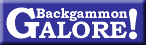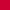Match EquitiesA Question Of Simplification Versus Accuracy Nigel Merrigan July 2000Over the past several years, there have a number of attempts to establish a more user-friendly approach to calculating match equities without burdening one's long-term memory with numerical junk.

One of the first to do this was a fellow by the name of Rick Janowski. He set upon the idea of converting Kit Woolsey's match equity table (from the book: How to Play Tournament Backgammon) to a more manageable formula. Others soon followed with their own ideas and methodologies. However, the solution to the 64,000-dollar question remained elusive: "How can we simplify without affecting the accuracy"?

In the article: "Calculating and using match equities", Steven Turner introduced the Turner formula which to a certain extent answered the call. In an 11-point match with a score of 9 to 6 (needs 2, needs 5) the Turner formula equates that the probability of the leader winning the match to be 73.4% with Woolsey's table giving 75%. Pretty close!

The Janowski formula gives 73.2%, also close.

After some serious math, a few trees worth of paper and a bowl of cornflakes, I managed to achieve 74.3 without spilling any milk. However, I needed more data before I could breakout the Kellog's again. With this in mind, I decided to compute all match equities (excluding Crawford) at match lengths ranging from 3 to 15-points. The purpose was to compare the deviations and percentage deviations of the Janowski, Turner and Merrigan formulas with Woolsey's match equity table. The results displayed are for each of the match lengths along with the overall results. See Table 1 below.

 Table 1: The sum of the total deviation and percentagedeviation at various match lengths
 Match 15 13 11 9 7 5 3 Total Dev% Woolsey Total 6770 4823 3218 1948 1009 386 60 0 0 Janowski Total 6757.5 4807.6 3202.6 1936.4 1000.2 381.3 59.4 Dev −12.55 −15.38 −15.36 −11.61 −8.78 −4.69 −0.6 −68.97 0.38 Dev% −0.185 0.32 0.48 0.6 −0.87 −1.22 −1 4.67 Turner Total 6894.6 4858.3 3213.5 1935.9 1001.2 384.8 61 Dev 124.64 35.32 −4.52 −12.1 −7.77 −1.2 1 186.55 1.02 Dev% 1.84 0.73 0.14 −0.62 −0.77 −0.31 1.67 6.08 Merrigan Total 6765.7 4829.8 3227.1 1956.1 1012.2 386.1 60.1 Dev −4.24 6.79 9.12 8.11 3.21 0.12 0.1 31.69 0.17 Dev% 0.06 0.14 0.28 0.42 0.32 0.03 0.17 1.42

To understand the table, lets consider the total sum of match equities in a 15-point match. Here, according to Kit Woolsey's table, the figure is 6770 compared to Rick's figure of 6757.5. Clearly, the deviation is −12.55 and the percentage deviation at any match score is −0.185. The total deviation for all match lengths is −68.97 with an overall percentage deviation of 4.67.

The end column shows the overall accuracy of Rick's formula at any match length (at any score) within the range of 3 to 15-points. This figure is 0.38 of a percent in either direction (+/−). Pretty good!

The Turner formula does not do too well in a 15-point match, but performs best at 11-points with a deviation of −4.52 and a percentage deviation of −0.14. The Merrigan formula performs best at all match lengths (with the exception of 11-points). In turn, the Merrigan formula achieves the best overall deviation of 31.69 and percentage deviation of 0.17. Clearly, the most accurate! However, how user-friendly is it? Let's take a look.

The formula is:

 50 + 92 − D6 + T × D

 D = the difference between the leader and trailer. T = the distance the trailer is away from winning the match.

To go back to our score of 9 to 6 in an 11-point match, the formula is computed as follows:

 D = 3, T = 5

 50 + 92 − 36 + 5 × 3 = 74.3%

Let's consider a score of 7 to 3:

 D = 4, T = 8

 50 + 92 − 46 + 8 × 4 = 75.2.  Woolsey has it as 75%

Looks simple enough, however I'll let you decide!

When Rick was developing the Janowski formula, he also considered those scores where the Crawford rule would be in effect. Hence he developed a formula for all Crawford scores, ranging from 3 to 15 points. This being the case, I decided to develop one of my own. So here it is!

 52 + 84 − D7 + T × T

The computational procedure is almost the same as the above formula, but with one exception. Instead of multiplying the difference between the leader and trailer, you simply multiply the distance the trailer requires to win the match. Consider a score of 10 to 6, Crawford.

 D = 4, T = 5

 52 + 84 − 47 + 5 × 4 = 85.3%.

Woolsey has it as 85%. Janowski is 86.4%.

Consider a score of 10 to 4,

 D = 6, T = 7

 52 + 84 − 67 + 7 × 6 = 90.9%.

Woolsey has it as 91%. Janowski is 91.6%.

It should not be ignored, that some of the credit should go to Rick Janowski as both formulas were based on his ideas and methodologies. Cheers Rick!!Return to:  Backgammon Galore